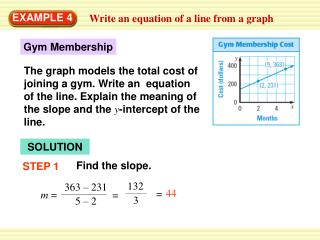Download PresentationEXAMPLE 4

# EXAMPLE 4

Download Presentation## EXAMPLE 4

- - - - - - - - - - - - - - - - - - - - - - - - - - - E N D - - - - - - - - - - - - - - - - - - - - - - - - - - -
##### Presentation Transcript

1. Gym Membership 363 – 231 44 = 5 – 2 The graph models the total cost of joining a gym. Write an equation of the line. Explain the meaning of the slope and the y-intercept of the line. Find the slope. STEP 1 132 = 3 EXAMPLE 4 Write an equation of a line from a graph SOLUTION m =

2. mx + b y = STEP 3 Write the equation. 231 = 44 2 + b = b 143 EXAMPLE 4 Write an equation of a line from a graph STEP 2 Find the y-intercept. Use the slope and one of the points on the graph. Use slope-intercept form. Substitute for x, y, and m. Simplify. Becausem = 44and b = 143, an equation of the line isy = 44x + 143.

3. STEP 1 Find the intercepts. 3x + 4y 3x + 4y = 12 = 12 3x +4 ( 0 ) 3 ( 0 ) + 4y = 12 = 12 EXAMPLE 5 Graph a line with equation in standard form Graph 3x + 4y = 12. SOLUTION The equation is in standard form, so you can use the intercepts. To find the x-intercept, let y = 0. To find the y-intercept, let x= 0. x = 4 y = 3

4. Graph the line. STEP 2 EXAMPLE 5 Graph a line with equation in standard form The intercepts are (4, 0) and (0, 3). Graph these points, then draw a line through the points.

5. The equation y = 50x + 125 models the total cost of joining a climbing gym. What are the meaning of the slope and the y-intercept of the line? ANSWER Slope: monthly feey- intercept: initial cost to join gym for Examples 4 and 5 GUIDED PRACTICE

6. 2x –3y = 6 ANSWER for Examples 4 and 5 GUIDED PRACTICE Graph the equation

7. ANSWER for Examples 4 and 5 GUIDED PRACTICE Graph the equation y = 4

8. ANSWER for Examples 4 and 5 GUIDED PRACTICE Graph the equation x = – 3